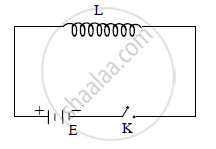Share

# Explain the Phenomenon of Self Induction. - Physics

#### Question

Explain the phenomenon of self induction

#### Solution

a. The phenomenon of production of an induced e.m.f in a coil due to change in current in the same coil is called self induction. It is also called as back-e.m.f.

b.Consider a coil connected with battery E, plug key K and inductor L carrying current of magnitude I as shown in figure.

c. Since magnetic flux linked with the coil is directly proportional to the current,
∴ φ ∝ I
∴ φ = LI .…(i)
where, L = constant called coefficient of self induction or self inductance of the coil, which depends upon the number of turns, shape, area of the coil and material of the core.

d. Induced e.m.f in the coil is given by,
e = -(dphi)/(dt)
e = − L (dI)/dt   ….(ii)
−ve sign in equation (ii) shows that self induced e.m.f opposes the rate of change of current.
∴ |e| = |-L (dI)/dt|

∴ |e|= L (dI)/dt
∴ Magnitude of self induced e.m.f is given by, e = L (dI)/dt
This is required induced e.m.f.

Is there an error in this question or solution?